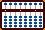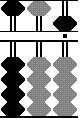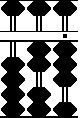###Tutorial: negative numbers from subtraction

Example: Subtract  0 - 95 = - 95  (negative 95)

##### Note: This is an advanced operation. Before tackling this, it's suggested you have a good working knowledge of the chapters Subtraction & Negative Numbers from Subtraction found in the online manual Abacus: Mystery of the Bead.

Step 1: Begin by clearing the soroban to start with 0 (zero)

#####Step 4

Step 2: Since we are subtracting a larger two digit number from a smaller one (in this case subtracting 95 from zero) begin by borrowing 1 from the hundreds column. Although this step can also be done mentally, for learning purposes I'll actually show it. Place 100 onto the soroban.

Step 3 & 4: Subtract 90 (step 3) and 5 (step 4) for a total of 95 from 100 just as you normally would. This leaves 5. Notice the grey beads (step 4) - they are the beads that don't have value. They add up to 94.  Following rules learned in the chapter Negative Numbers from Subtraction in the online manaul Abacus: Mystery of the Bead mentally add 1 grey bead to the last rod that has value. This leaves the answer  -95 (negative 95).

#### REMEMBER TO GIVE BACK IF A NEGATIVE ANSWER BECOMES POSITIVE IN A SUBSEQUENT OPERATION

Lets take this one step further and add a larger positive number to the negative answer. This will yield a positive answer.

Example: -95 + 118 = 23

#####Step 6

Step 5: Add 118 to the negative answer on the soroban leaving 123. Since we borrowed 1 from the hundreds column in an earlier step we have to give it back if a subsequent operation yields a positive answer.

Step 6 and the answer: Give back the 100 borrowed in the earlier operation. This leaves 23, the correct answer.